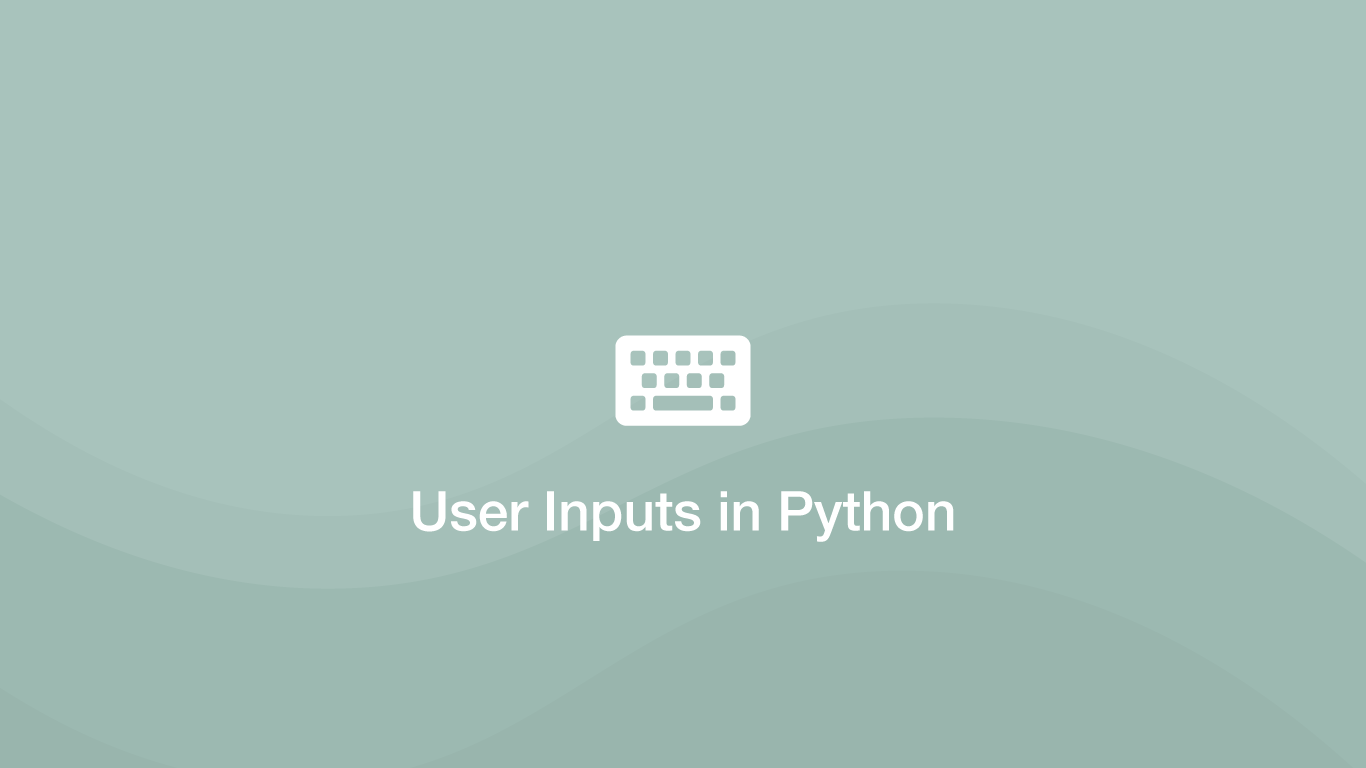# How to Get Inputs in PythonSometimes we need to ask a user to input data into a program at certain stages of its operation. We can achieve this with the Python `input()` function, which can be used to display an input prompt to a user with a custom message.

In this tutorial, we will learn how to use the Python `input()` function with examples.

## How to use the input() Function

To use the `input()` function, pass the message to send to the user inside the `()` (parenthesis) of `input()` and store the result in a variable. Let's create a new file called `hello.py` containing a function that will ask the user to input their name, which will then be returned back to them saying hello.

``````vim hello.py
``````
``````def hello():
print(f'Hi {name}!')

hello()
``````

Now the program can run by typing `python` followed by the file name from the terminal and the user will be prompted to type their name in:

``````python hello.py
``````
``````Please enter your name: john
``````
``````Hi john!
``````

## Getting Numbers from input()

It is import to be aware that `input()` will bring numerical inputs from the user into Python as strings. To convert a string to a number in Python use the `int()` function. In the example below, we will evaluate that the string has indeed been converted into a number by using the type() function.

``````def number():
number = input('Please enter a number: ')
print(type(int(number)))

number()
``````
``````python number.py
``````
``````Please enter a number: 3
``````
``````<class 'int'>
``````

## input_raw()

In older versions of python (2.*) you will need to use the `raw_input()` function to prompt users for input.

``````def hello():
print('Hi %s' % (name))

hello()
``````

## Conclusion

You now know how to prompt users for data at any point in your program and provide a custom message in the prompt.

#### Related Tutorials### How to Execute Shell Commands in Python

September 22, 2020### How to Use Argparse in Python

September 25, 2020### How to Convert a String to a Number in Python

October 01, 2020### How to Calculate Mean, Median, Mode and Range in Python

October 07, 2020### How to Get the Value of an Input Text Box in JavaScript or jQuery

December 18, 2020### How to Create and Use Lists (Arrays) in Python

September 01, 2020